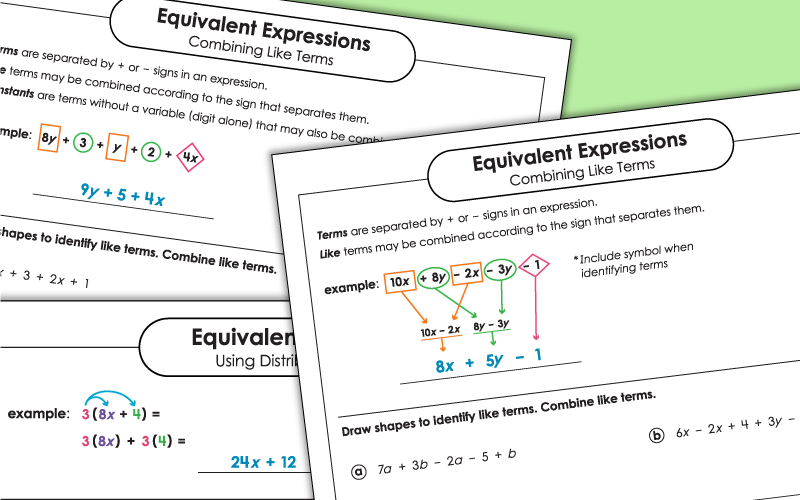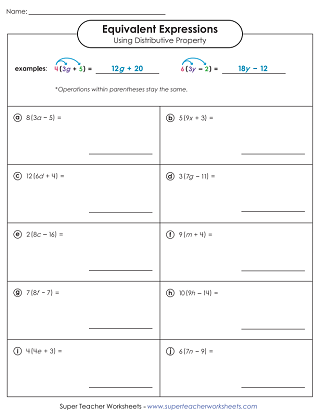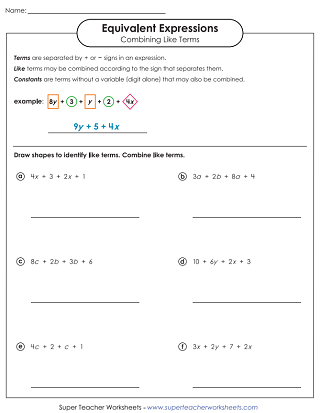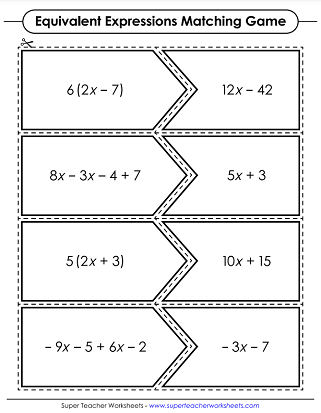# Equivalent Algebraic Expressions

With these printable worksheets, students will practice simplifying and finding equivalent algebraic expressions. Most of these worksheets align with CCSS.6.EE.3 and/or CCSS.7.EE.A.1.## Combining Like Terms(Simplifying Expressions)

Combine like terms to simplify each expression. This basic level includes only addition (no subtraction).
Here is another worksheet to use for practice combining like terms for basic algebraic expressions.
Simplify each expression by combining like terms. The intermediate level include addition and subtraction.
Use this worksheet to help students practice combining like terms in algebraic expressions with both addition and subtraction.
Combine like terms to generate a new algebraic expression. This advanced version includes addition, subtraction, and exponents.
Create new expressions by combining like terms. This is an advanced-level worksheet.
Combine the like terms to create an equivalent expression. These expressions include addition, subtraction, and exponents.

## Finding Equivalent Expressions Using Distributive Property

Use your knowledge of the distributive property to find equivalent expressions.
ex: 5(4y+6) = 5(4y) + 5(6) = 20y+30
Use the distributive property to find equivalent expressions. In this intermediate-level worksheets, students are simplifying expressions with parenthesis.
ex: 5(3a-5) = 24a-40

## Mixed Types

Cut out the cards. Students match equivalent expressions. These cards include some that require students to use the distributive property and some that do not.
For each of these task cards, students will answer a question about equivalent expressions. Some require distributive property, some do not.
More Algebra Topics

STW has a variety of algebra and pre-algebra worksheet topics. From this page, you'll be able to link to resources on evaluating expressions, writing expressions, one and two-step equations, inequalities, and more.

## Sample Worksheet ImagesMy Account
Site Information